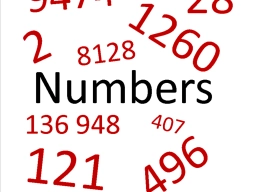# The sum 30

The sum of 8 times a number and 5 times another number is 184. The first number minus the second number is -3. What are the numbers?

a =  13
b =  16

### Step-by-step explanation:Did you find an error or inaccuracy? Feel free to write us. Thank you!

Tips for related online calculators
Do you have a linear equation or system of equations and looking for its solution? Or do you have a quadratic equation?